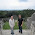## Arduino Controlled V-Amp Pro - Part 2

So I threw out all of the code from the last post. Can't take the bull by the horns like that.

## Testing

First off, I set the V-Amp to communicate through the MIDI in on channel 1. Consult the manual on how to do this. Editing the settings on that piece of gear is like working a Chinese puzzle box.

Upon examining the existing hardware, it was determined that the code has to use reverse HIGH/LOW logic. So when a switch goes low, instead of being normally high, it will change effects/effect banks and make the negative end of an LED go low when necessary. Get all that?

## Test Program 1 – Bank Up/Bank Down

Like the title suggests, this code just changes the number (bank number) on the V-Amp's 7 segment displays using 2 momentary buttons. There are 5 effects per bank.
See image for hardware required.

```int program = 0x00; int bank = 0x01; int number_in_bank = 0x05; void setup() {   for(int i = 2; i <= 3; i++)   {     pinMode(i, INPUT);   }     //  Set MIDI baud rate:   Serial.begin(31250);   changeProgram(program); } void loop() {     if(digitalRead(2) == LOW){     program += number_in_bank;     changeProgram(program);     delay(500);   }   if(digitalRead(3) == LOW){     program -= number_in_bank;     changeProgram(program);     delay(500);   } } void changeProgram(int program) {   Serial.print(0xC0, BYTE);   Serial.print(program, BYTE); }```

Verdict: WORKS!

## Test Program 2 – One whole switchboard

This code will utilize one whole switchboard from the FCB1010. The button hooked into pin 2 of the Arduino will move the effects up one bank (add 5).
See image for hardware required.

``` int program = 0x00; int bank = 0x00; int number_in_bank = 0x05; void setup() {   for(int i = 2; i <= 7; i++)   {     pinMode(i, INPUT);   }     //  Set MIDI baud rate:   Serial.begin(31250);   changeProgram(program); } void loop() {     if(digitalRead(2) == LOW)   {     program += number_in_bank;     bank += number_in_bank;     changeProgram(program);     delay(500);   }   else if(digitalRead(7) == LOW)   {     program = bank + 0x00;     changeProgram(program);     delay(500);   }   else if(digitalRead(6) == LOW)   {     program = bank + 0x01;     changeProgram(program);     delay(500);   }   else if(digitalRead(5) == LOW)   {     program = bank + 0x02;     changeProgram(program);     delay(500);   }   else if(digitalRead(4) == LOW)   {     program = bank + 0x03;     changeProgram(program);     delay(500);   }   else if(digitalRead(3) == LOW)   {     program = bank + 0x04;     changeProgram(program);     delay(500);   } } void changeProgram(int program) {   Serial.print(0xC0, BYTE);   Serial.print(program, BYTE); }```

Verdict: I tried factoring out the repetitive code into an elegant loop. It just plain didn't work. As written: WORKS!

## Test 3 – LEDs

Factored-out some code. Also, the FCB1010 has LEDs that light up that correspond with whatever effect is active. This has to be accounted for in the code.

See image for hardware required.``` int program = 0x00; int bank = 0x00; int number_in_bank = 0x05; void setup() {   for(int i = 2; i <= 7; i++)   {     pinMode(i, INPUT);   }  for(int i = 8; i <= 12; i++)  {    pinMode(i, OUTPUT);    digitalWrite(i, HIGH);  }   //  Set MIDI baud rate:   Serial.begin(31250);   changeProgram(program,0x00,0); } void loop() {     if(digitalRead(2) == LOW)   {     program += number_in_bank;     bank += number_in_bank;     changeProgram(program,0x00,0);     delay(500);   }   else if(digitalRead(7) == LOW)   {     changeProgram(program,0x00,12);   }   else if(digitalRead(6) == LOW)   {     changeProgram(program, 0x01,11);   }   else if(digitalRead(5) == LOW)   {     changeProgram(program,0x02,10);   }   else if(digitalRead(4) == LOW)   {     changeProgram(program, 0x03, 9);   }   else if(digitalRead(3) == LOW)   {     changeProgram(program,0x04,8);   } } void changeProgram(int program, int effect_number, int LED) {   program = bank + effect_number;   Serial.print(0xC0, BYTE);   Serial.print(program, BYTE);   digitalWrite(LED,LOW);     for(int i = 8; i <= 12; i++){     if(i != LED)     {       digitalWrite(i, HIGH);     }   }   delay(500); }```

Verdict: WORKS!!!!!!1!!one!!!

## Future Installments

I ran out of I/O and I lack the know-how to build a multiplexing circuit. As such, I will have to wait until the Arduino MEGA256 arrives to add more stuff. (Sad face)

Still to go: add another 5 effects changing switches and a down bank switch (Another footswitch board), expression pedals and (optional) an LED/LCD screen telling me what effect is active.
<- Part 1       Part 4 ->
Categories: , , , ,

1.2.